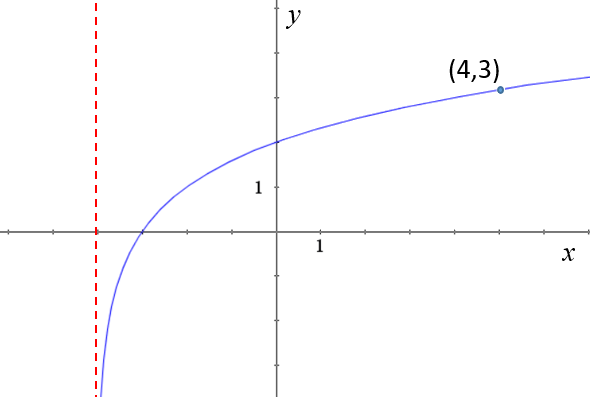# Exam-Style Questions on Inverse Functions

## Problems on Inverse Functions adapted from questions set in previous Mathematics exams.

### 1.

IB Standard

Part of the graph of $$f(x) = {\log _b}(x + 4)$$ for $$x > - 4$$ is shown below.The graph passes through A(4, 3) , has an x-intercept at (-3, 0) and has an asymptote at $$x = - 4$$.

(a) Find the value of $$b$$.

The graph of $$f(x)$$ is reflected in the line $$y = x$$ to give the graph of $$g(x)$$.

(b) Write down the y-intercept of the graph of $$g(x)$$.

(c) Sketch the graph of $$g(x)$$, noting clearly any asymptotes and the image of A.

(d) Find $$g(x)$$ in terms of $$x$$.

If you would like space on the right of the question to write out the solution try this Thinning Feature. It will collapse the text into the left half of your screen but large diagrams will remain unchanged.

The exam-style questions appearing on this site are based on those set in previous examinations (or sample assessment papers for future examinations) by the major examination boards. The wording, diagrams and figures used in these questions have been changed from the originals so that students can have fresh, relevant problem solving practice even if they have previously worked through the related exam paper.

The solutions to the questions on this website are only available to those who have a Transum Subscription.

Exam-Style Questions Main Page

Search for exam-style questions containing a particular word or phrase:

To search the entire Transum website use the search box in the grey area below.